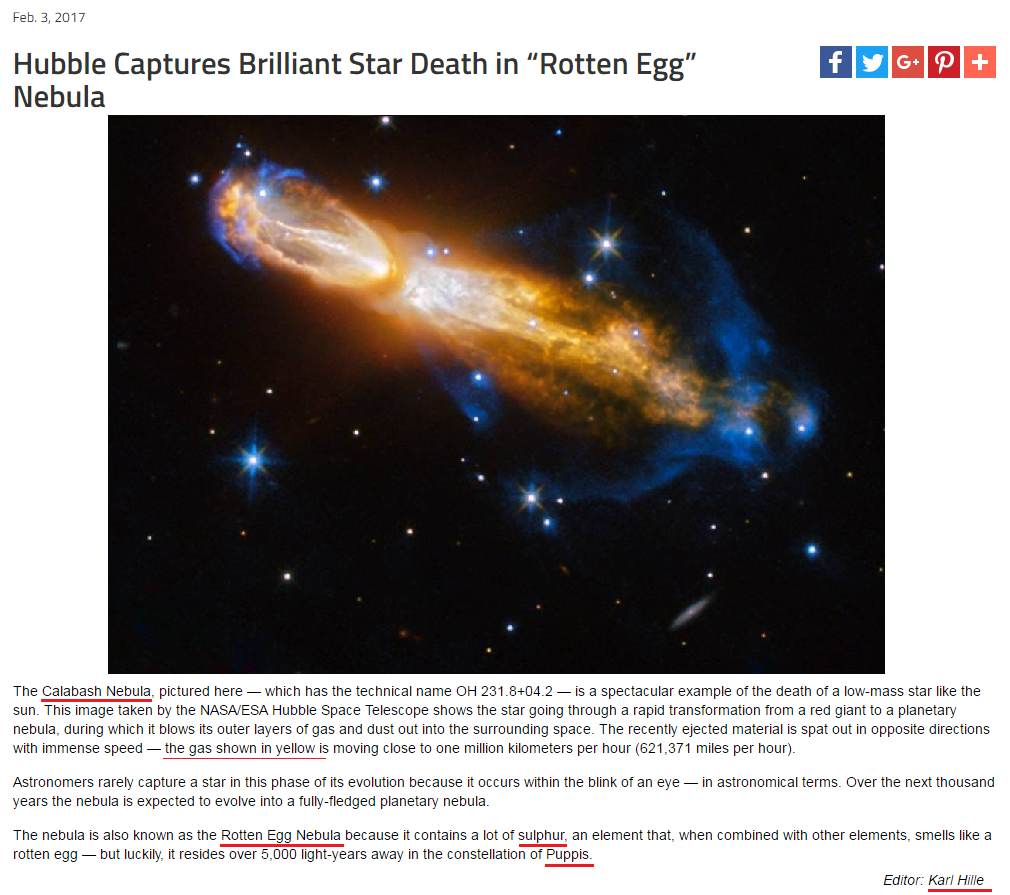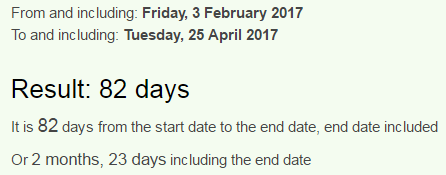## Cipher## Feb 5, 2017

### Hubble Captures Brilliant photoshoped “Rotten Egg” Nebula

This story came out on 3rd of February -
Even CNN has a post on this joke -  http://edition.cnn.com/2017/02/07/world/hubble-star-death-nebula-trnd/index.htmlFebruary 3rd is written 2/3 in the US
Hubble = 8+3+2+2+3+5 = 23 (Reduced)

February Third = 6+5+2+9+3+1+9+7 + 2+8+9+9+4 = 74 (Reduced)
We are receiving picture of Calabash Nebula ( Calabash -- Cabalash -- Kaballah? )
Calabash = 3+1+12+1+2+1+19+8 = 47 (Ordinal)
Picture is supposedly taken from "HST" - initials for Hubble Space Telescope
HST = 8+19+20 = 47 (Ordinal)
47th prime number is 211 and the title connects as well
Hubble Captures Brilliant Star Death in Rotten Egg Nebula = 211 (Reduced)
Also in the story they talk about "yellow gas" shown in the fully made up image
yellow gas = 7+5+3+3+6+5 + 7+1+10 = 47 (S Exception)
yellow gas = 25+5+12+12+15+23 + 7+1+19 = 119 (Ordinal)

Calabash Nebula = 3+1+3+1+2+1+10+8 + 5+5+2+3+3+1 = 48 (S Exception)
Rotten Egg = 9+6+2+2+5+5 + 5+7+7 = 48 (Reduced)
Rotten Egg = 108+90+120+120+30+84 + 30+42+42 = 666 (Sumerian)

48th prime number is 223 and this joke of a story comes 2 months 23 days before 27th anniversary of deployment of Hubble "telescope"
Editor of the story has 223 name gematria
Karl Hille = 20+1+90+30 + 8+9+30+30+5 = 223 (English)Also it comes 107 days before 5/20/2017 -  27th anniversary since HST 'entered service' (3 months, 18 days is interesting showing up with 107, in the bible Jesus catches 153 fish in John 21:11)
318th prime number is 2111 and 107 is 153 in octal system (just like 17th triangular number)In the post they give us this "technical name OH 231.8+04.2 (we can see multiple things here, 153 in octal system is 231 which also connects to the 231.8... then 4.2 might be related to the date numerology) - before the number we see "OH" = 23, which might be connecting to "Hubble" = 23

This seems mostly connected to 20th of May with 318 coding
2/3/2017 - 2 + 3 + 20 + 17 = 42
5/20/17 - 5 + 20 + 17 = 42
Rotten Egg Nebula = 9+6+2+2+5+5 + 5+7+7 + 5+5+2+3+3+1 = 67 (Reduced)
Twentieth of May = 2+5+5+5+2+9+5+2+8 + 6+6 + 4+1+7 = 67 (Reduced)

"The nebula is also known as the Rotten Egg Nebula because it contains a lot of sulphur, an element that, when combined with other elements, smells like a rotten egg — but luckily, it resides over 5,000 light-years away in the constellation of Puppis."
Puppis = 7+3+7+7+9+1 = 34 (Reduced)
sulphur = 1+3+3+7+8+3+9 = 34 (Reduced)
The story comes on 34th day of the year and the editor of the story has matching reduced gematria

The last part of the post after the double dash is interesting as well, they have a habit of finishing their posts with 666 but this time it is 343 (7x7x7)
but luckily it resides over lightyears away in the constellation of Puppis = 343 (S Exception)

In CNN's post they call it "Stinky star"
stinky star = 19+20+9+14+11+25 + 19+20+1+18 = 156 (Ordinal)
thirty three = 20+8+9+18+20+25 + 20+8+18+5+5 = 156 (Ordinal)